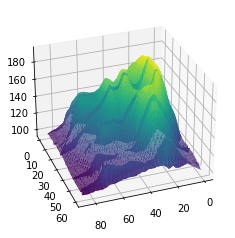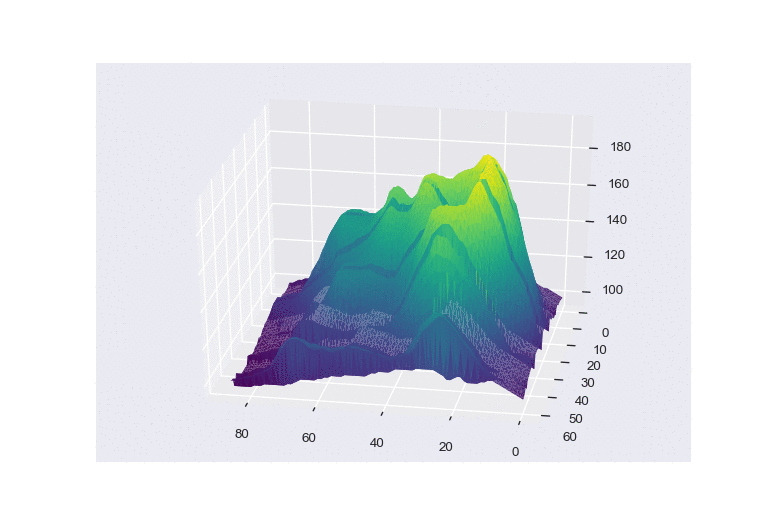# Animated 3D chart

This blogpost explains how to build a 3d surface area with `matplotlib`. It also shows how to vary the camera angle to produce an animation using `Image Magick`.

## 📍 Basic 3d density chart

Let's start by making a basic 3d density chart. This step is described in its own blogpost here, so let's just remember how the code looks like:

``````# library
from mpl_toolkits.mplot3d import Axes3D
import matplotlib.pyplot as plt
import pandas as pd
import seaborn as sns

# Get the data (csv file is hosted on the web)
url = 'https://raw.githubusercontent.com/holtzy/The-Python-Graph-Gallery/master/static/data/volcano.csv'

# Transform it to a long format
df=data.unstack().reset_index()
df.columns=["X","Y","Z"]

# And transform the old column name in something numeric
df['X']=pd.Categorical(df['X'])
df['X']=df['X'].cat.codes

# Make the plot
fig = plt.figure()
ax = fig.gca(projection='3d')
ax.plot_trisurf(df['Y'], df['X'], df['Z'], cmap=plt.cm.viridis, linewidth=0.2)

# Set the angle of the camera
ax.view_init(30,70)
``````## 🔄 Rotate the camera

The last line of the code chunck above is of high interest here. It set the angle the camera uses to display the figure (`70°` here).

To produce an animation, we're gonna produce several figure with varying angles, and output them at `.png` format. This is done thanks to a `for` loop:

``````# library
from mpl_toolkits.mplot3d import Axes3D
import matplotlib.pyplot as plt
import pandas as pd
import seaborn as sns

# Get the data (csv file is hosted on the web)
url = 'https://raw.githubusercontent.com/holtzy/The-Python-Graph-Gallery/master/static/data/volcano.csv'

# Transform it to a long format
df=data.unstack().reset_index()
df.columns=["X","Y","Z"]

# And transform the old column name in something numeric
df['X']=pd.Categorical(df['X'])
df['X']=df['X'].cat.codes

# We are going to do 20 plots, for 20 different angles
for angle in range(70,210,2):

# Turn interactive plotting off
plt.ioff()

# Make the plot
fig = plt.figure()
ax = fig.gca(projection='3d')
ax.plot_trisurf(df['Y'], df['X'], df['Z'], cmap=plt.cm.viridis, linewidth=0.2)

# Set the angle of the camera
ax.view_init(30,angle)

# Save it
filename='/Users/yan.holtz/Desktop/Volcano_step'+str(angle)+'.png'
plt.savefig(fname=filename, dpi=96)
plt.gca()
plt.close(fig)
``````

Now, you should have a set of 12 images in the specified folder (`/Users/yan.holtz/Desktop/` for me). `Image magick` is a command line tool that allows to concatenate those images in a `gif` file.

## 🎦 Animation

Install ImageMagick with this line of bash: `brew install imagemagick`

If you don't have `brew` installed, visit the image magick homepage for explanations.

Once the tool is installed, you can concatenate the 12 images using the following command:

``````# Bash
# convert -delay 80 Volcano_step*.png animated_volcano.gif
``````

Note: the above line of code is written in `bash`, not in `python`. You have to execute it in a terminal, not in your python environment.

And here is the final result## Contact & Edit

👋 This document is a work by Yan Holtz. Any feedback is highly encouraged. You can fill an issue on Github, drop me a message onTwitter, or send an email pasting `yan.holtz.data` with `gmail.com`.